a. Only one common root

Let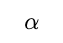be the common root of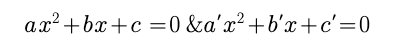then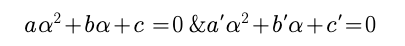By Cramer's Rule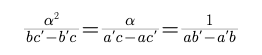Therefore ,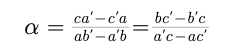So the condition for a common root is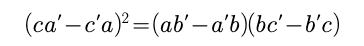b.

If both roots are same then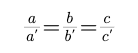Example :

Find p and q such that px2 +5x+2=0 and 3x2 +10x+q=0. have both roots in common?

Solution :

a1=p,b1=5,c1=2

a2 = 3, b2 = 10, c2 = q

We know that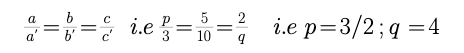Post By : Rahul Kumar 08 Apr, 2020 2943 views Maths